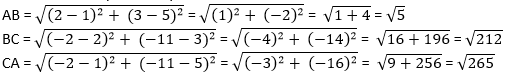Newbie

# Determine if the points (1, 5), (2, 3) and (-2, -11) are collinear. Q.3

• 0

What is the best solution of coordinate geometry  of class 10 ,give me easy way to solve this question quickly . Determine if the points (1, 5), (2, 3) and (-2, -11) are collinear.

Share

1. The sum of the lengths of any two line segments is equal to the length of the third line segment then all three points are collinear.

Consider, A = (1, 5) B = (2, 3) and C = (-2, -11)

Find the distance between points; say AB, BC and CASince AB + BC ≠ CA

Therefore, the points (1, 5), (2, 3), and ( – 2, – 11) are not collinear.

• 0